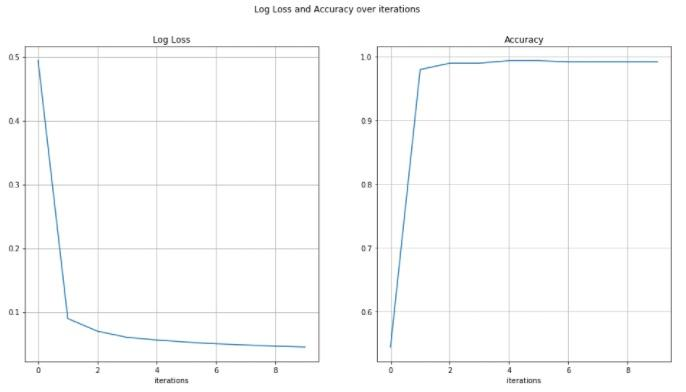Need help? We are here

# USE Jupyter Notebook Given Code: ‘Part of answer’ but incomplete…..

USE Jupyter Notebook

Given Code: ‘Part of answer’ but incomplete…..

``# Importing librariesimport pandas as pdimport numpy as npfrom sklearn.neighbors import KNeighborsClassifierfrom sklearn.tree import DecisionTreeClassifierfrom sklearn.model_selection import train_test_splitfrom sklearn import metrics# Loading the datasetdata = pd.read_csv('diabetes.csv')# Separating features and targetfeatures = data[['Pregnancies', 'Glucose', 'BloodPressure', 'SkinThickness', 'Insulin', 'BMI', 'DiabetesPedigreeFunction', 'Age']]target = data['Outcome']# Splitting training and testing data in the ratio 70 : 30 respectivelyx_train, x_test, y_train, y_test = train_test_split(features, target, random_state=0, test_size=0.3)# KNN modelmodelKNN = KNeighborsClassifier()# Train the modelmodelKNN.fit(x_train, y_train)# Predict test sety_pred_knn = modelKNN.predict(x_test)# Print accuracy of the KNN modelprint(modelKNN.score(x_test,y_test))# Print Confusion matrixprint(metrics.confusion_matrix(y_test, y_pred_knn))# Print Classification Report for determining precision, recall, f1-score and support of the modelprint(metrics.classification_report(y_test, y_pred_knn))# Decision Tree modelmodelDT = DecisionTreeClassifier()# Train the modelmodelDT.fit(x_train, y_train)# Predict test sety_pred_dt = modelDT.predict(x_test)# Print accuracy of the Decision Tree modelprint(modelDT.score(x_test,y_test))# Print Confusion matrixprint(metrics.confusion_matrix(y_test, y_pred_dt))# Print Classification Report for determining precision, recall, f1-score and support of the modelprint(metrics.classification_report(y_test, y_pred_dt))``

Please do the data cleaning and feature selection…

Please use the given code/layout given below original question….

ORIGINAL QUESTION:

“”for more info check original .ipynb file””

Fill out the code below so that it creates a multi-layer perceptron with a single hidden layer (with 4 nodes) and trains it via back-propagation. Specifically your code should:

1. Initialize the weights to random values between -1 and 1
2. Perform the feed-forward computation
3. Compute the loss function
4. Calculate the gradients for all the weights via back-propagation
5. Update the weight matrices (using a learning_rate parameter)
6. Execute steps 2-5 for a fixed number of iterations
7. Plot the accuracies and log loss and observe how they change over time

First, initialise the parameters. This means determining the following:

Size of the network

Hidden layers (or a single hidden layer in this example) can be any size. Layers with more neurons are more powerful, but also more likely to overfit, and take longer to train. The output layer size corresponds to the number of classes.

Number of iterations

This parameter determines how many times the network will be updated.

Learning rate

Each time we update the weights, we do so by taking a step into the direction that we calculated will improve the accuracy of the network. The size of that step is determined by the learning rate. Taking small steps will slow the process down, but taking steps that are too large can cause results to vary wildly and not reach a stable optimum.

Next, fill in the code below to train a multi-layer perceptron and see if it correctly classies the input.

in[]# Reshape y
y =

# Initializing weights

W_1 =
W_2 =

# Definining number of iterations and learning rate(‘lr’)
num_iter =

learning rate =

in[]# Creating empty lists for loss values(error) and accuracy
loss_vals, accuracies = [], []

for j in range(num_iter):
# Do a forward pass through the dataset and compute the loss

# Decide on intervals and add on the current loss and accuracy to the respective list

# Update the weights

in[]# Plot the loss values and accuracyHigher number of iterations and lower learning rate seems to improve accuracy